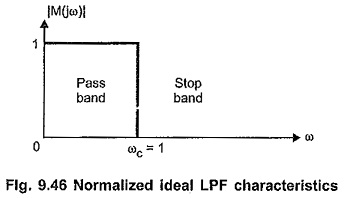## Normalized Low Pass Filter Characteristics:

Normalized Low Pass Filter Characteristics – The passive filters are the filters consisting only passive components such as resistors, inductors, capacitors. These classical filters are designed starting with filter networks such as T type, Π type etc. Low Pass Filter Characteristics is obtained by connecting inductor in the series arm and capacitor in the shunt arm of the network, while high pass filter is obtained by connecting capacitor in the series arm and inductor in the shunt arm of the network. These filters are designed for given cut-off frequency and characteristic impedance.

There are few disadvantages of these filters as follows.

1. Attenuation does not rise sharply with frequency in the stop band.
2. In pass band, the characteristic impedance Z0 varies with frequency so proper termination of filter is not possible.

In comparison with classical filter design, modern filter design approach is exactly other-way. In modern filter design we start with required characteristics and then try to synthesize a network giving desired characteristics. Let us study modern filter design approach based on approximation theory.

Low Pass Filter (LPF) can be converted in any other type of filter. So let us study low pass filter characteristics first. Consider ideal LPF characteristics as shown in Fig. 9.46.The response shown in Fig. 9.46 is normalized response in which we assume ωc = 1 rad/sec and response in pass band |M(jω)| = 1. Then by using proper scale factors for magnitude and frequency, we can get desired filter characteristics.

But this ideal response is not possible with any of the practical filter network. Hence it is desired to have a characteristics which approximates the ideal characteristics shown in Fig. 9.46. Many scientists have suggested many approximations to the ideal characteristics.

Scroll to Top